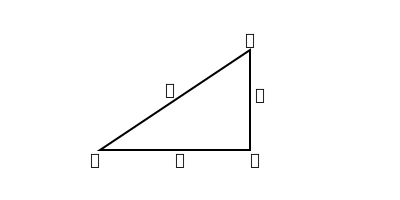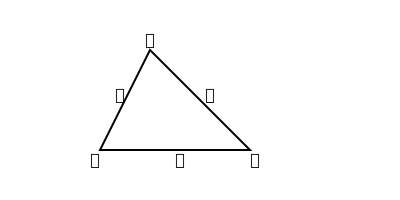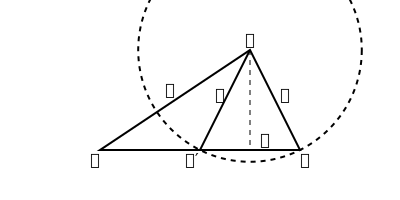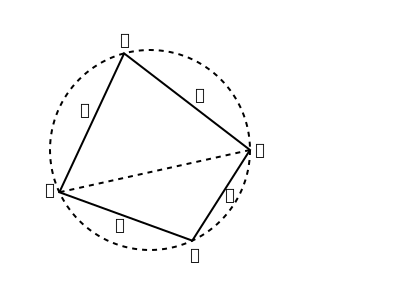output.to from Sideway
Draft for Information Only

# Content

`Plane Trigonometry Solution of Triangles Scalene Triangles  Case I  The Ambiguous Case  Case II  Case III Quadrilateral Inscribed in a Circle Sources and References`

# Plane Trigonometry

## Solution of Triangles

718Right-angled triangles are solved by formula 𝑐2=𝑎2+𝑏2 719 {𝑎=𝑐sin𝐴𝑏=𝑐cos𝐴𝑎=𝑏tan𝐴

## Scalene Triangles

720### Case I

The equation 𝑎sin𝐴=𝑏sin𝐵701 will determine any one of the four quantities 𝐴, 𝐵, 𝑎, 𝑏 when the remaining three are known.

### The Ambiguous Case

721When, in Case I, two sides and an acute angle opposite to one of them are given, we have,, from the figure, sin𝐶=𝑐sin𝐴𝑎 Then 𝐶 and 180−𝐶 are the vlues of 𝐶 and 𝐶′, by (622). Also 𝑏=𝑐cos𝐴±𝑎2−𝑐2sin2𝐴 because 𝑏=𝐴𝐷±𝐷𝐶 722 When an angle 𝐵 is to be determined from the equation sin𝐵=𝑏𝑎sin𝐴 and 𝑏𝑎 is a small fraction; the circular measure of 𝐵 may be approximated to by putting sin(𝐵+𝐶) for sin𝐴, and using theorem (796). 723

### Case II

When two sides 𝑏, 𝑐 and the included angle 𝐴 are known, the third side 𝑎 is given by the formula 𝑎2=𝑏2+𝑐2−2𝑏𝑐cos𝐴702 when logarithms are not used. 724 Otherwise, employ the following formula with logarithms, tan𝐵−𝐶2=𝑏−𝑐𝑏+𝑐cot𝐴2 725 Obtained from 𝑏−𝑐𝑏+𝑐=sin𝐵−sin𝐶sin𝐵+sin𝐶(701), and then applying (670) and (671).
𝐵+𝐶2 having been found from the above equation, and 𝐵+𝐶2 being equal to 90°−𝐴2, we have 𝐵=𝐵+𝐶2+𝐵−𝐶2, 𝐶=𝐵+𝐶2𝐵−𝐶2 𝐵 and 𝐶 having been determined 𝑎 can be found by Case I. 726 If the logarithms of 𝑏 and 𝑐 are known, the trouble of taking out log(𝑏−𝑐) and log(𝑏+𝑐) may be avoided by employing the subsidiary angle 𝜃=tan−1𝑏𝑐, and the formula 727 tan12(𝐵−𝐶)=tan𝜃−𝜋4cot𝐴2655 728 Or else the subsidiary angle 𝜃=cos−1𝑐𝑏, and the formula tan12(𝐵−𝐶)=tan2𝜃2cot𝐴2643 729 𝑎=(𝑏+𝑐)sin𝐴2cos12(𝐵−𝐶)From the figure in 960, by drawing a perpendicular from 𝐵 to 𝐸𝐶 produced. 730 If 𝑎 be required in terms of 𝑏, 𝑐 and 𝐴 alone, and in a form adapted to logarithmic computation, employ the subsidiary angle 𝜃=sin−14𝑏𝑐(𝑏+𝑐)2cos2𝐴2 and the formula 𝑎=(𝑏+𝑐)cos𝜃702, 637

### Case III

When the three sides are known, the angles may be found without employing logarithms, from the formula 731 cos𝐴=𝑏2+𝑐2−𝑎22𝑏𝑐703 732 If logarithms are to be used, take the formula for sin𝐴2, cos𝐴2, tan𝐴2, (704), and (705).

## Quadrilateral Inscribed in a Circle733 cos𝐵=𝑎2+𝑏2−𝑐2−𝑑22(𝑎𝑏+𝑐𝑑) From 𝐴𝐶2=𝑎2+𝑏2−2𝑎𝑏cos𝐵=𝑐2+𝑑2+2𝑐𝑑cos𝐵, by (702), and 𝐵+𝐷=180°. 734 sin𝐵=2𝑄𝑎𝑏+𝑐𝑑613, 733 735 𝑄=(𝑠−𝑎)(𝑠−𝑏)(𝑠−𝑐)(𝑠−𝑑)=area of 𝐴𝐵𝐶𝐷 and 𝑠=12(𝑎+𝑏+𝑐+𝑑) Area=12𝑎𝑏sin𝐵+12𝑐𝑑sin𝐵; substitute sin𝐵 from last. 736 𝐴𝐶2=(𝑎𝑐+𝑏𝑑)(𝑎𝑑+𝑏𝑐)(𝑎𝑏+𝑐𝑑)702, 733 737 Radius of circumscribed circle =14𝑄(𝑎𝑏+𝑐𝑑)(𝑎𝑐+𝑏𝑑)(𝑎𝑑+𝑏𝑐)713, 734, 736 738 if 𝐴𝐷 bizect the side of the triangle 𝐴𝐵𝐶 in 𝐷, tan𝐵𝐷𝐴=4△𝑏2−𝑐2 739 cot𝐵𝐴𝐷=2cot𝐴+cot𝐵 740 𝐴𝐷2=14(𝑏2+𝑐2+2𝑏𝑐cos𝐴)=12(𝑏2+𝑐212𝑎2) 742 If 𝐴𝐷 bisect the angle 𝐴 of a triangle 𝐴𝐵𝐶, tan𝐵𝐷𝐴=cot𝐵−𝐶2=𝑏+𝑐𝑏−𝑐tan𝐴2 743 𝐴𝐷=2𝑏𝑐𝑏+𝑐cos𝐴2 744 𝐴𝐷=𝑏𝑐sin𝐴𝑎=𝑏2sin𝐶+𝑐2sin𝐵𝑏+𝑐 745 𝐵𝐷∼𝐶𝐷=𝑏2−𝑐2𝑎=𝑎tan𝐵−tan𝐶tan𝐵+tan𝐶

## Sources and References

https://archive.org/details/synopsis-of-elementary-results-in-pure-and-applied-mathematics-pdfdrive

ID: 210900007 Last Updated: 9/7/2021 Revision: 0 Ref:References

1. B. Joseph, 1978, University Mathematics: A Textbook for Students of Science &amp; Engineering
2. Ayres, F. JR, Moyer, R.E., 1999, Schaum's Outlines: Trigonometry
3. Hopkings, W., 1833, Elements of TrigonometryHome 5

Management

HBR 3

Information

Recreation

Culture

Chinese 1097

English 337

Computer

Hardware 154

Software

Application 207

Latex 35

Manim 203

Numeric 19

Programming

Web 285

Unicode 504

HTML 65

CSS 63

SVG 9

ASP.NET 240

OS 422

Python 64

Knowledge

Mathematics

Algebra 84

Trigonometry 31

Geometry 32

Calculus 67

Engineering

Mechanical

Rigid Bodies

Statics 92

Dynamics 37

Control

Natural Sciences

Electric 27